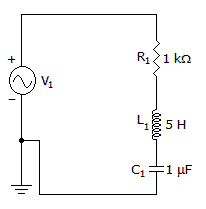# Electronics - RLC Circuits and Resonance

## Why Electronics RLC Circuits and Resonance?

In this section you can learn and practice Electronics Questions based on "RLC Circuits and Resonance" and improve your skills in order to face the interview, competitive examination and various entrance test (CAT, GATE, GRE, MAT, Bank Exam, Railway Exam etc.) with full confidence.

## Where can I get Electronics RLC Circuits and Resonance questions and answers with explanation?

IndiaBIX provides you lots of fully solved Electronics (RLC Circuits and Resonance) questions and answers with Explanation. Solved examples with detailed answer description, explanation are given and it would be easy to understand. All students, freshers can download Electronics RLC Circuits and Resonance quiz questions with answers as PDF files and eBooks.

## Where can I get Electronics RLC Circuits and Resonance Interview Questions and Answers (objective type, multiple choice)?

Here you can find objective type Electronics RLC Circuits and Resonance questions and answers for interview and entrance examination. Multiple choice and true or false type questions are also provided.

## How to solve Electronics RLC Circuits and Resonance problems?

You can easily solve all kind of Electronics questions based on RLC Circuits and Resonance by practicing the objective type exercises given below, also get shortcut methods to solve Electronics RLC Circuits and Resonance problems.

### Exercise :: RLC Circuits and Resonance - General Questions

1.

What is the applied voltage for a series RLC circuit when IT = 3 mA, VL = 30 V, VC = 18 V, and R = 1000 ohms?

 A. 3.00 V B. 12.37 V C. 34.98 V D. 48.00 V

Explanation:

No answer description available for this question. Let us discuss.

2.

What is the bandwidth of the circuit?A. 31.8 Hz B. 32.3 Hz C. 142 Hz D. 7.2 kHz

Explanation:

No answer description available for this question. Let us discuss.

3.

In a parallel RLC circuit, which value may always be used as a vector reference?

 A. current B. reactance C. resistance D. voltage

Explanation:

No answer description available for this question. Let us discuss.

4.

How much current will flow in a 100 Hz series RLC circuit if VS = 20 V, RT = 66 ohms, and XT = 47 ohms?

 A. 1.05 A B. 303 mA C. 247 mA D. 107 mA

Explanation:

No answer description available for this question. Let us discuss.

5.

What is the Q (Quality factor) of a series circuit that resonates at 6 kHz, has equal reactance of 4 kilo-ohms each, and a resistor value of 50 ohms?

 A. 0.001 B. 50 C. 80 D. 4